Question
show all work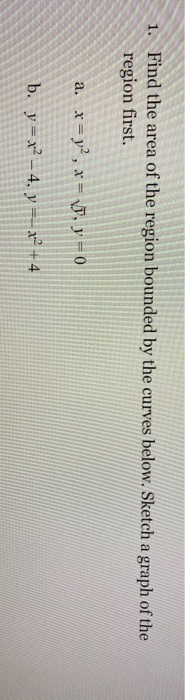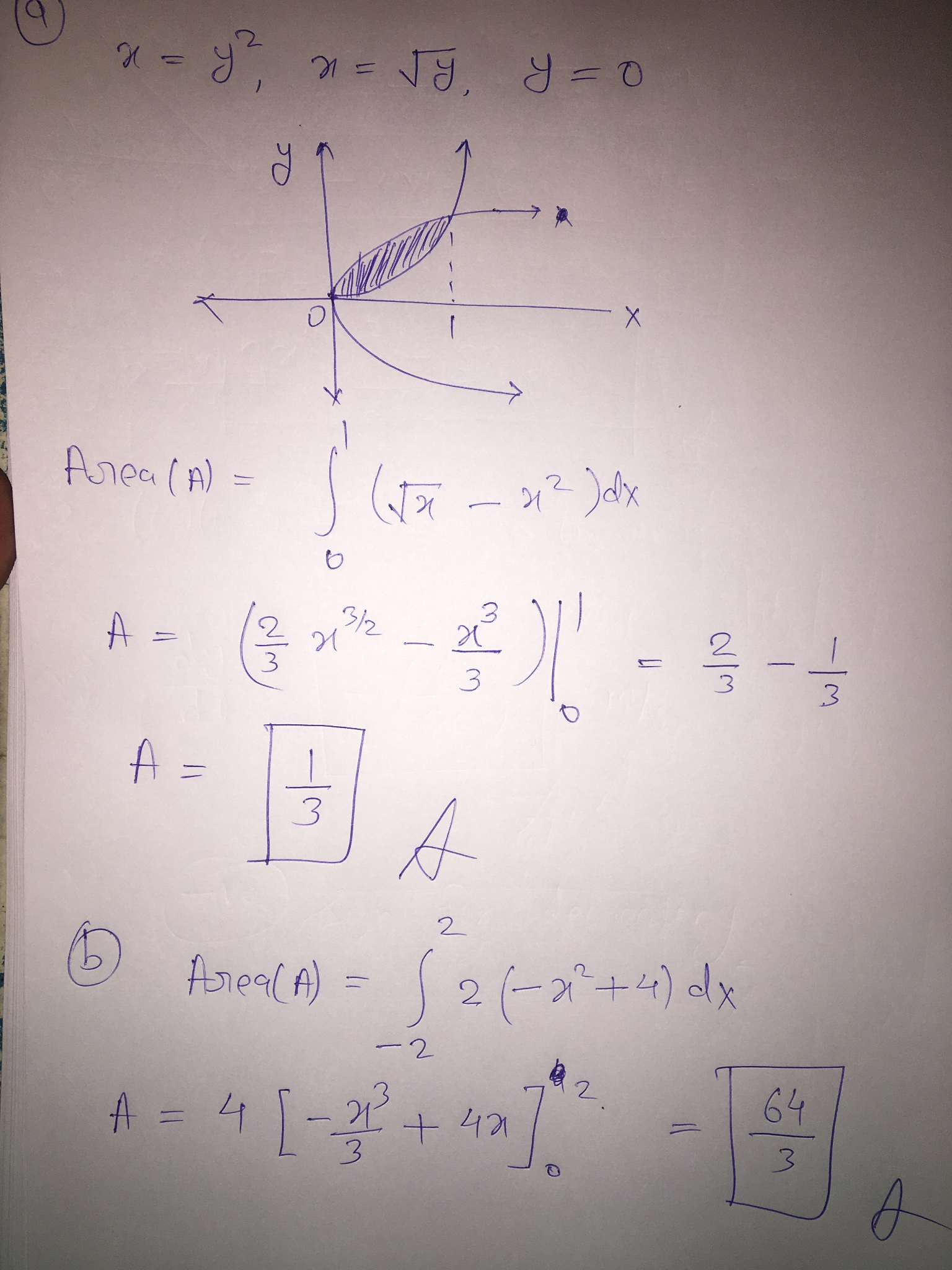#### Earn Coins

Coins can be redeemed for fabulous gifts.

Similar Homework Help Questions
• ### Home Work Task 1 Total M Determine the area of the region enclosed by the two...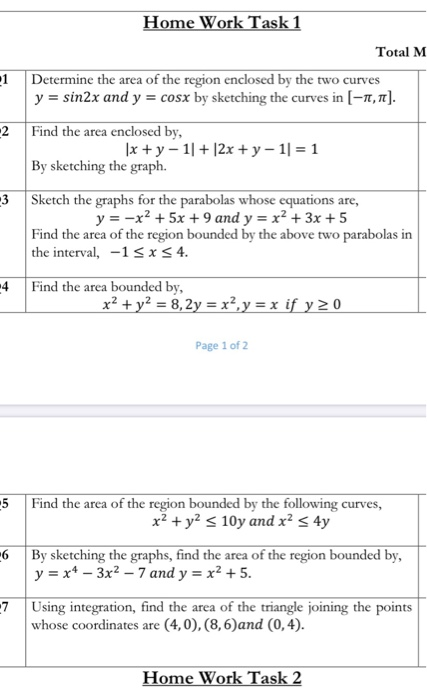Home Work Task 1 Total M Determine the area of the region enclosed by the two curves y = sin2x and y = cosx by sketching the curves in (-1,1]. Find the area enclosed by, 1x + y - 11 + 12x + y - 11 = 1 By sketching the graph. 3 Sketch the graphs for the parabolas whose equations are, y = -x2 + 5x + 9 and y = x² + 3x + 5 Find the area...

• ###  Sketch the region bounded above the curve of y = x2 - 6, below y...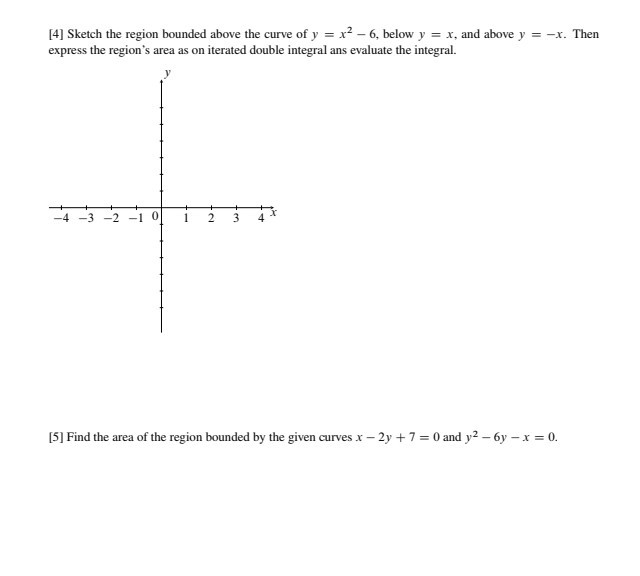Sketch the region bounded above the curve of y = x2 - 6, below y = x, and above y = -x. Then express the region's area as on iterated double integral ans evaluate the integral. -4 -3 -2 -1 0 1 2 3 4  Find the area of the region bounded by the given curves x - 2y + 7 = 0 and y2 -6y - x = 0.

• ### Find the area of the following region Sketch the bounding curves and the mopon in question...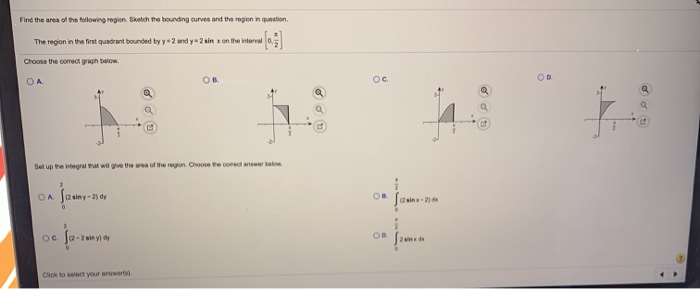Find the area of the following region Sketch the bounding curves and the mopon in question The region in the fint quadrant bounded by y2 and y-2sin on the interval Choose the correct graph below OA OB OC OD Set up to Wegral hat will give the sea of the region. Choose the corect answer below OA 12 siny-2) dy OB sin-21 oc. Jaz 22 - 2 single OD 2 Click to set your ar Find the area of the...

• ### Show all work so that I can follow your thought process 1) Area between curves Determine the area of the region bounded by the following two functions: 2) Use the region bounded by the curves to d...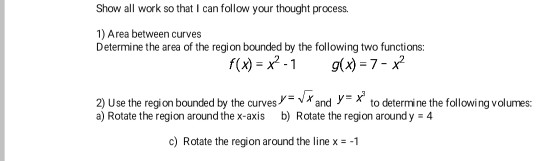Show all work so that I can follow your thought process 1) Area between curves Determine the area of the region bounded by the following two functions: 2) Use the region bounded by the curves to determine the following volumes: a) Rotate the region around the x-axis b) Rotate the region aroundy 4 c) Rotate the region around the line x-1 Show all work so that I can follow your thought process 1) Area between curves Determine the area of...

• ### 1. (25 points) Find the area of the region bounded by the given curves by two...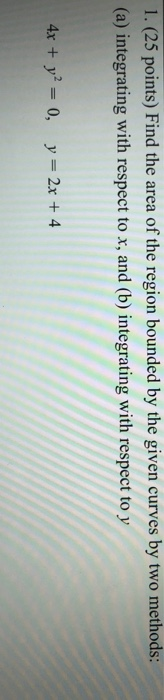1. (25 points) Find the area of the region bounded by the given curves by two methods: (a) integrating with respect to x, and (b) integrating with respect to y 4x + y2 = 0, y = 2x + 4

• ### Find the area of the region between curves       1. Find Find the area of...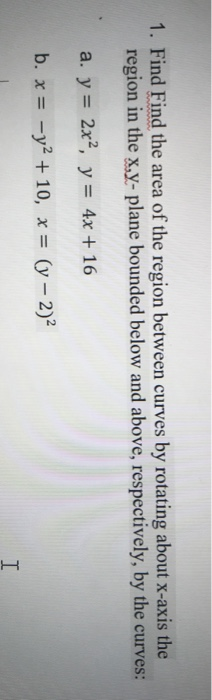Find the area of the region between curves       1. Find Find the area of the region between curves by rotating about x-axis the region in the x,y- plane bounded below and above, respectively, by the curves: a. y = 2x2, y = 4x + 16 b. x = -y2 + 10, x = (y – 2) I

• ### Please show all work 1. Find the area of the region bounded by the graphs of...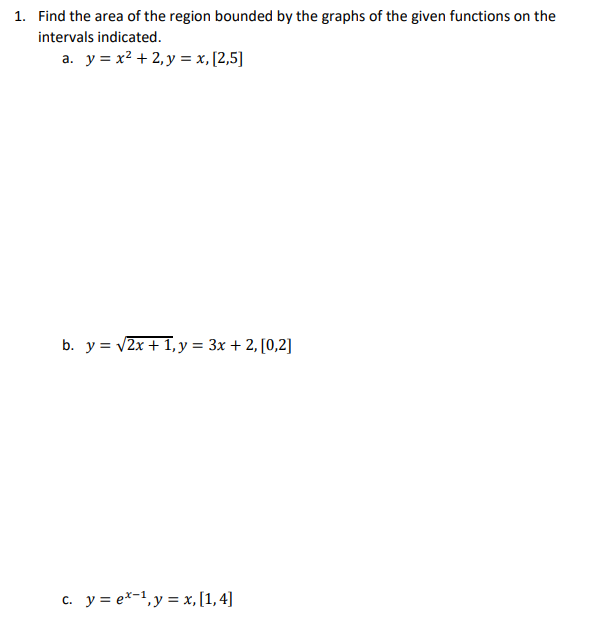Please show all work 1. Find the area of the region bounded by the graphs of the given functions on the intervals indicated. a. y = x2 + 2, y = x, (2,5) b. y = (2x +1, y = 3x + 2, [0,2] C. y = ex-1, y = x,[1,4]

• ### show all work please (5 pts) Find the area of the region bounded by the graphs...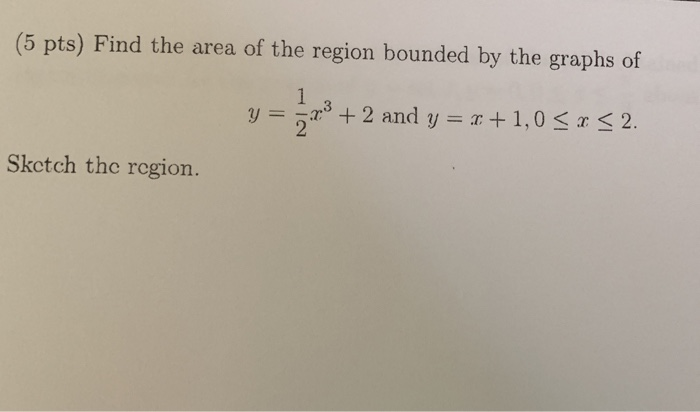show all work please (5 pts) Find the area of the region bounded by the graphs of y + 2 and y = [ +1,0 < x < 2. 2 Sketch the region.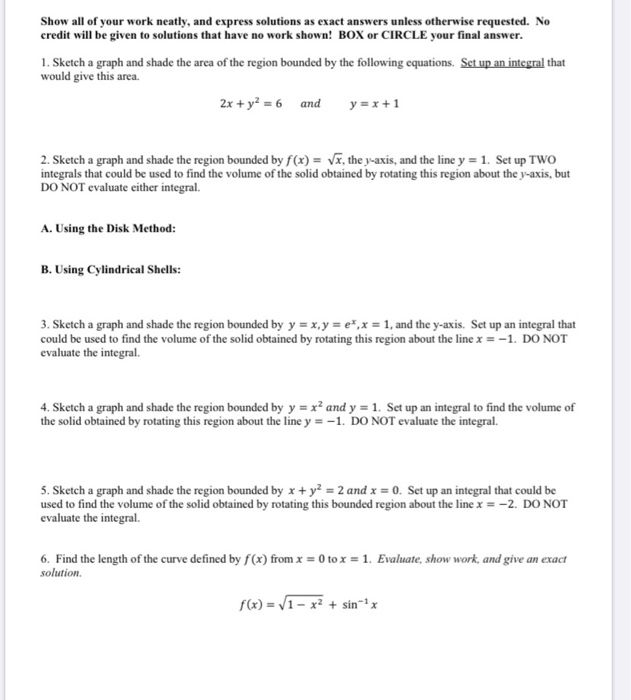Please help with 1-10 and please show all work thanks. Show all of your work neatly, and express solutions as exact answers unless otherwise requested. No credit will be given to solutions that have no work shown! BOX or CIRCLE your final answer. 1. Sketch a graph and shade the area of the region bounded by the following equations. Set up an integral that would give this area. 2x + y2 = 6 and y=x+1 2. Sketch a graph and...

• ### Show all work please. 1Find the area of the region bounded by the curves y 2x2...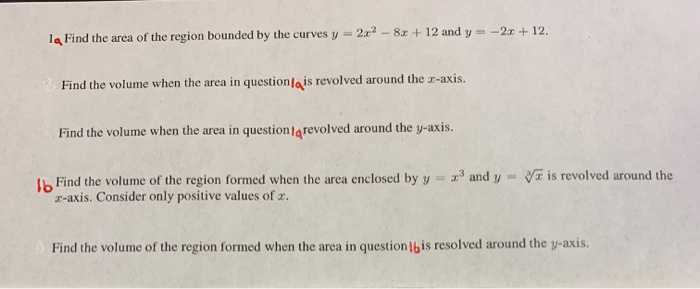Show all work please. 1Find the area of the region bounded by the curves y 2x2 - 8x + 12 and y=-2 + 12. Find the volume when the area in question/qis revolved around the 3-axis. Find the volume when the area in question/grevolved around the y-axis. Va is revolved around the Ib Find the volume of the region formed when the area enclosed by y = x3 and y 2-axis. Consider only positive values of x. Find the volume...